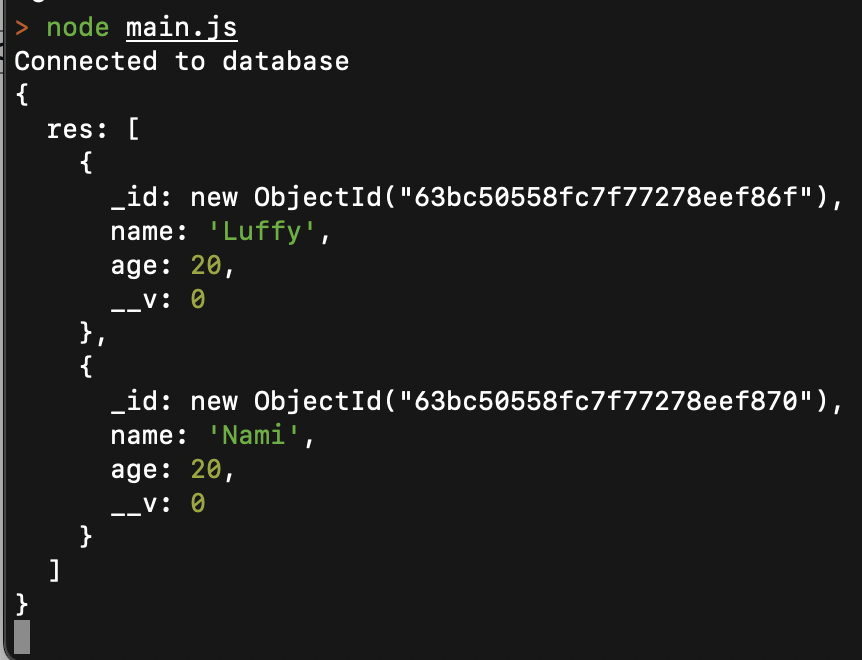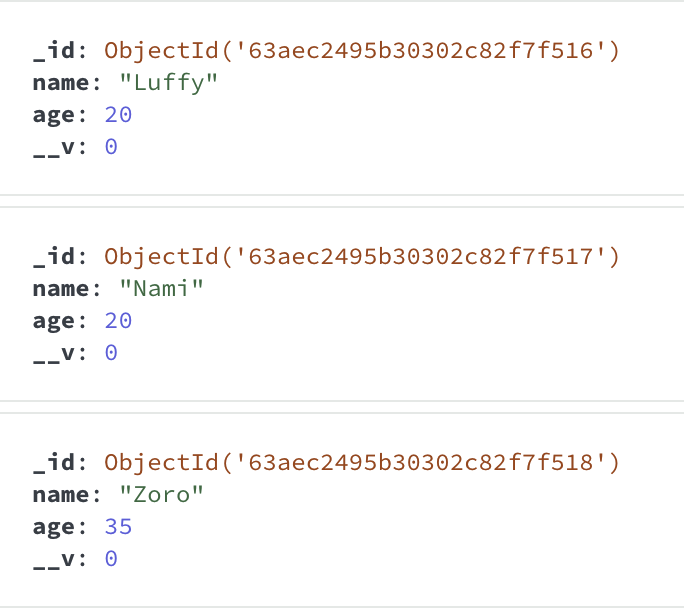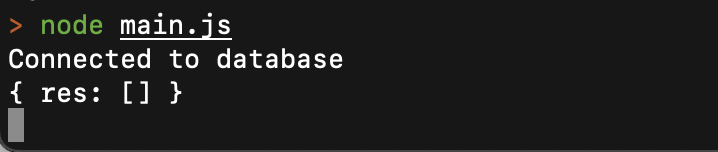Open in App
Not now

# Mongoose Query.prototype.lte() API

• Last Updated : 27 Mar, 2023

The Mongoose Query API lte() method is used to scan all the documents in a collection and return those whose path values are less than or equal to those passed in the method’s argument.

Syntax:

`Query.prototype.lte( path, val )`

Parameters: It accepts the following parameters as mentioned above and described below:

• path: It is a string that identifies the attribute name in the mongoose schema
• val: It is a number that determines the maximum value to compare from all the documents’ path values

Return type: It returns a Query object as a response.

Creating node application And Installing Mongoose:

Step 1: Create a node application using the following command:

```mkdir folder_name
cd folder_name
npm init -y
touch main.js```

Step 2: After completing the Node.js application, Install the required module using the following command:

`npm install mongoose`

Example 1: In this example, we will use this method to return documents that have age less than or equal to “20”

Filename: main.js

## Javascript

 `// Importing the module` `const mongoose = require(``'mongoose'``)`   `// Creating the connection` `mongoose.connect(``'mongodb://localhost:27017/query-helpers'``,` `    ``{` `        ``dbName: ``'event_db'``,` `        ``useNewUrlParser: ``true``,` `        ``useUnifiedTopology: ``true` `    ``}, err => err ? console.log(err)` `        ``: console.log(``'Connected to database'``));`   `let personSchema = ``new` `mongoose.Schema({` `    ``name: {` `        ``type: String,` `    ``},` `    ``age: {` `        ``type: Number,` `    ``}` `});`   `let personsArray = [` `    ``{` `        ``name: ``'Luffy'``,` `        ``age: 20` `    ``},` `    ``{` `        ``name: ``'Nami'``,` `        ``age: 20,` `    ``},` `    ``{` `        ``name: ``'Zoro'``,` `        ``age: 35` `    ``}` `]`   `let Person = mongoose.model(``'Person'``, personSchema);`   `(async () => {` `    ``await Person.insertMany(personsArray)` `    ``const res = await Person.find().where(``'age'``).lte(20);` `    ``console.log({ res });` `})();`

Step to Run Application: Run the application using the following command from the root directory of the project:

`node main.js`

OutputGUI Representation of the  Database using MongoDB Compass:Example 2: In this example, we will use this method to return documents that have an age less than or equal to “18”

Filename: main.js

## Javascript

 `// Importing the module` `const mongoose = require(``'mongoose'``)`   `// Creating the connection` `mongoose.connect(``'mongodb://localhost:27017/query-helpers'``,` `    ``{` `        ``dbName: ``'event_db'``,` `        ``useNewUrlParser: ``true``,` `        ``useUnifiedTopology: ``true` `    ``}, err => err ? console.log(err)` `        ``: console.log(``'Connected to database'``));`   `let personSchema = ``new` `mongoose.Schema({` `    ``name: {` `        ``type: String,` `    ``},` `    ``age: {` `        ``type: Number,` `    ``}` `});`   `let personsArray = [` `    ``{` `        ``name: ``'Luffy'``,` `        ``age: 20` `    ``},` `    ``{` `        ``name: ``'Nami'``,` `        ``age: 20,` `    ``},` `    ``{` `        ``name: ``'Zoro'``,` `        ``age: 35` `    ``}` `]`   `let Person = mongoose.model(``'Person'``, personSchema);`   `(async () => {` `    ``await Person.insertMany(personsArray)` `    ``const res = await Person.find().where(``'age'``).lte(18);` `    ``console.log({ res });` `})();`

Step to Run Application: Run the application using the following command from the root directory of the project:

`node main.js`

Output:GUI Representation of the  Database using MongoDB Compass:My Personal Notes arrow_drop_up
Related Articles